# RL — Meta-Learning

So far, AI cannot learn as efficiently as a human. Many classifiers take millions of training samples to train and knowledge is not shared among tasks. Each task is trained independently from others. In this article, we will look into the problems and then check out some proposed solutions.

What is the problem?

Comparing with a human, there are two critical weaknesses:

1. Sample efficiency: Deep learning has poor sample efficiency. For example, to recognize digit handwriting, we typically read 6000 samples per digit.
2. Poor transferability. We don’t learn from previous experience or knowledge.

Meta-learning

So what is meta-learning? We define it as “learn how to learn”. Personally, I don’t think this clarifies anything. In reality, we don’t know the exact definition or the solution yet. So it is still a loose term referring different approaches. In this article, we will focus on the following areas:

• recurrent models,
• meta-optimization, and
• metric learning.

# Few-Shot Learning

But let’s define some basic concept first. In CIFAR-10, we have 60,000 images in ten different categories. In few-shot learning, we train a model with a far larger amount of tasks but far fewer samples per task. Our ultimate goal is generalizing knowledge and apply the model to new tasks that even not train before.

For example, in task 1, we are given 3 samples to learn emoji. Then, with a new emoji, we train the model to associate it with one of the previous samples.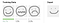Meta-learning program Reptile from OpenAI

In our second task, we train it with the alphabets.

We repeat the process many times with different tasks. Once the training is done, we measure the model versatility by testing it with a task that we have not performed before, distinguishing the Chinese characters. And yet, the model can associate the testing samples correctly with the new input.

We may wonder what is the difference between few-shot training and our traditional DL using a large dataset. In DL, we use regularization to make sure we are not overfitting our model with a small dataset. But by training the model with so many samples and iterations, we are overfitting our tasks. What we learned cannot be generalized to other tasks.

Let me demonstrate some of the constraints in DL. We often get stuck when we test samples that are not common in the dataset. For example, in the toy category, there are way too many variants that a DL model can learn. As shown below, the yellow toy duck is badly classified. In few-shot training, the key objective is to process data that we have not been trained before.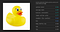Mistaken the duck toy as a savings bank or a musical instrument

In a one-shot training, we will only provide one training sample per category. In the example below, the training contains multiple datasets. Each dataset contains a 1-shot, 5-class classification task, i.e. five samples from five different classes. Then the next dataset are for 5 different categories.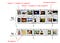Modified from source

In this one-shot training, we can train a RNN to learn the training data and labels. When we present a test input, we should predict its label correctly. (We will come back on this later.)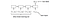Source

In meta-testing, we provide datasets that never trained before. In this example, the key focus for meta-learning is to learn the secret in classifying objects. Once we have learned from hundred tasks, we would not focus on individual classes. Instead, we should discover the general knowledge in classifying objects. So even when we are presented with classes never seen before, we should manage to solve the problem.

If we collect tasks smarter, we learn better.

Omniglot

Before we go into the details. Let’s introduce Omniglot. It is a popular dataset for the few-shot learning. The following are 20 drawings from Omniglot that represent 20 classes.

# Recurrent Models

The first meta-learning approach is the recurrent model. We feed data into an RNN-like model to remember what we see so far. When we are present with a testing input, we recall from memory to tell us what it is. However, we don’t have enough memory for everything we see. The recurrent model store features and we use linear algebra similar to word embedding to correlate information.

Let’s do a recap in a memory network (MN) first. MN uses a controller to extract features from the input. Then we use the features to access memory.

For example, you take a phone call but you cannot recognize the voice immediately. The voice sounds a whole lot like your cousin (0.7 chance) but it also resembles the voice of your elder brother (0.3 chance). In the diagram above, each row represents an object. We compute a weight w for each row to measure its relevancy with the input. Then we computed a weighted sum from all rows as the memory output. For classification problems, we can fit the output to a classifier to predict its class.

(If you want more details, take a quick look at Neural Turing Machine later.)

Memory-Augmented Neural Networks is one of the meta-learning methods using an external memory network with RNN. In supervised learning, we provide both input and label at the same time step t. However, in this model, the label is not provided until the next timestamp t+1(shown below). This is a technique to discourage the RNN cell to map the input to the class label directly. We want our model to memorize experience.

Training Memory-Augmented Neural Networks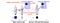Modified from source

In Memory-Augmented Neural Networks, we use external memory to store sample representation and class label information. A controller, typically implemented as LSTM, produces a key from the input which is either stored in the external memory or used to retrieve a particular memory. The whole system is then trained with backpropagation.

If we can learn from experience, we learn better.

After the first episode, the samples will be shuffled and the model will start establishing memory in predicting labels.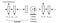source

For details, we recommend readers to read the original paper.

# Learning optimizers

In the second meta-learning approach, we optimize the model more efficiently. After the training of each task, we can use the information to update the model.

Yet, we are learning a particular task rather than finding the fundamental knowledge behind all the learned tasks. So instead of updating the model immediately, we wait until a batch of tasks is completed. We later merge all we learned from these tasks for a single update. We combine the reward gradient for each newly suggested parameters learned from each task. Then we perform the backpropagation. This approach fulfills the concept of “learn what we learn”.

The Model-agnostic meta-learning (MAML) utilizes the concept above to update models. It is simple and it is almost the same as our traditional DL gradient descent with one added line of code. Here, we do not update the model parameters immediately after each task. Instead, we wait until a batch of tasks to be completed.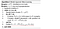Source

For each task, we use backpropagation to compute a suggested model.

We then consolidate the losses of the trained tasks and backpropagate the losses to make the next model update:

But in this step, we sample new task τᵢ from τ to compute the loss. Conceptually, we are finding a model that minimizes the losses of the tasks.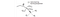Source

Graphically, each task may drive the model parameters into different directions. Introducing the meta-learning step and the few-shot datasets, we learn a model that works with many tasks but not overfit with a particular one.

There are other optimizers with the objective of learning more efficiently. For example, OpenAI proposes another optimizer call Reptile. In stochastic gradient descent, we compute one gradient descent and update the model. Then we fetch the next batch of data for the next iteration. In Reptile, it performs multiple-step gradient descent for each task and uses the result in the last step to update the model with a concept similar to the running mean.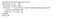Source

In the OpenAI paper, it demonstrates mathematically why both MAML and Reptile behave similarly. In short, it argues that MAML uses

But we will let you to read the original paper for the arguments.

If we optimize better, we learn better.

# Metric learning

The third meta-learning approach we will discuss is the metric learning. Do you remember picture pixel-by-pixel? No. To learn, we need to extract the maximum information we care with the least amount of memory. So, the third meta-learning approach focuses on how well we extract features but not overdo it. In Siamese Neural Networks (the figure below), we use two identical networks sharing the same model parameter values to extract features for two samples. Then we feed the extracted features into a discriminator to identify whether both samples belong to the same class of object. For example, we can compute its cosine similarity (p) of their feature vectors. If they are similar, p should be close to 1. Otherwise, they should be close to 0. Based on the labels of the samples and p, we train the networks accordingly. In short, we want to find features that make samples belong to the same class or differentiate them.

There is another method called Matching Network that is very similar to the Siamese Neural Networks.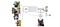Source

g and f are feature extractors using deep networks to extract features for our input and our testing (the German Shepard) samples. Usually, g and f are the same and share the same deep network. Then we compare their similarity and use a softmax function to compute whether they are similar. Once again, we compute a cost function from our prediction to train our feature extractor. Here is the mathematical formalization:

If we know how to represent data better, we learn better.

# Other approaches

There are other meta-learning approaches focus on how to tune a model with better hyperparameters. i.e. Learn how to tune the hyperparameters smartly. Alternatively, we can combine DL layers to form a new model dynamically.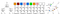source

These methods make models more accurate but not necessary more efficient in learning with fewer samples. So we will not discuss further in our meta-learning discussion.

# Thoughts

Learn how to learn better is not a challenge for the machines only but for the human also. Meta-learning has been studied for decades but yet we do not fully understand how it is done. To close our thoughts, here is the possible area of study to improve learning efficiency.

• Collect better information to learn.
• Learn from past experiences better.
• Know better how to represent information.
• How to optimize (solve) model better.
• Explore better.
• Associate better.
• Generalize better.

Deep Learning